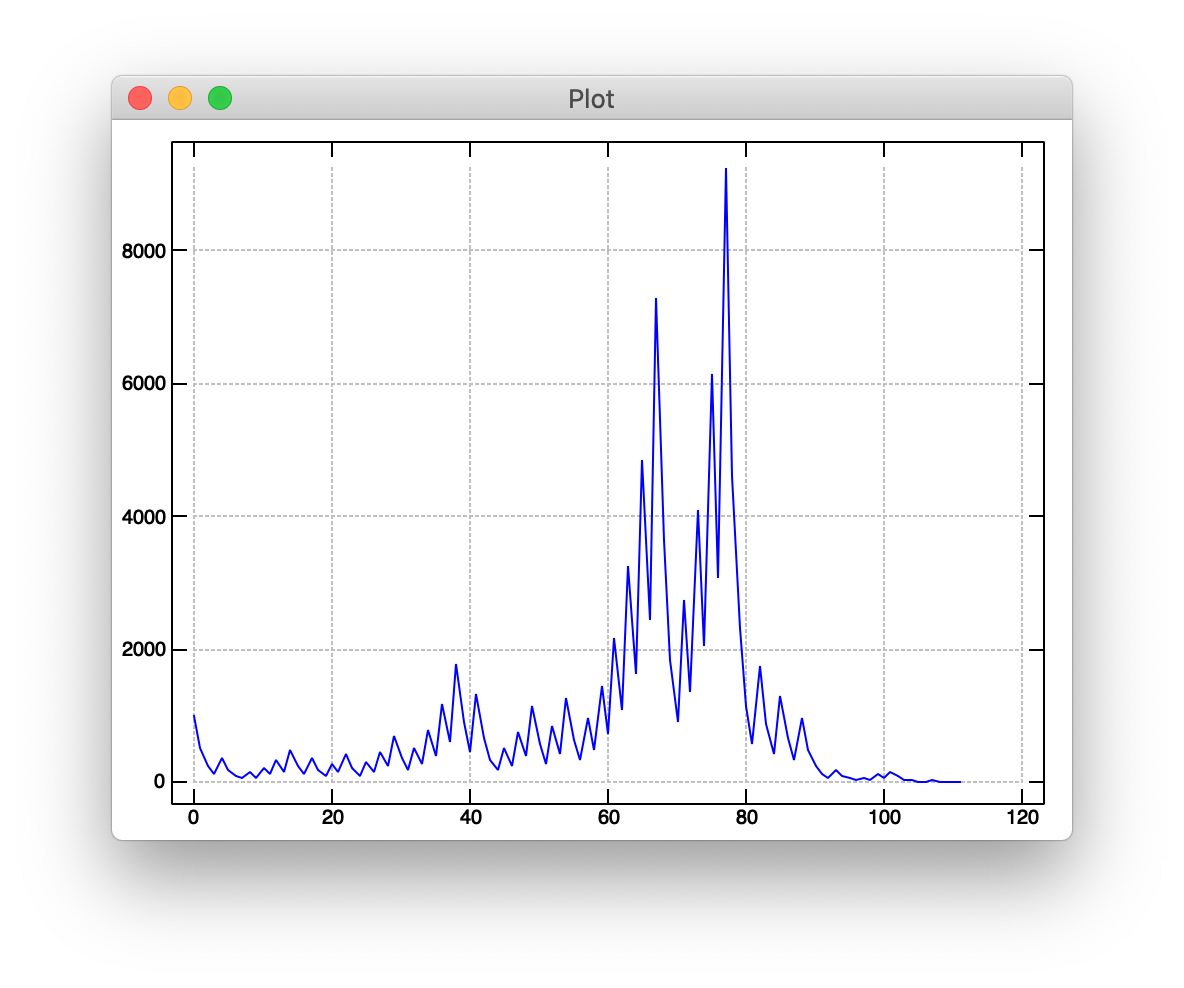I’ve been playing around quite a bit with the Collatz Conjecture after watching the latest Coding Train video on the subject. In an effort to keep my J muscles from atrophying, I decided to use the language to implement a Collatz sequence generator.

At its heart, the Collatz sequence is a conditional expression. If the current number is even, the next number in the sequence is the current number divided by two. If the current number is odd, the next number in the sequence is one plus the current number multiplied by three:We can write each of these branches with some straight-forward J code. Our even case is simply the divide (`%`) verb bonded (`&`) to `2`:

``````
even =: %&2
``````

And our odd case is a “monadic noun fork” of `1` plus (`+`) `3` bonded (`&`) to multiply (`*`):

``````
odd =: 1 + 3&*
``````

We can tie together (```) those two verbs and use agenda (`@.`) to pick which one to call based on whether the argument is even:

``````
next =: even`odd@.pick
``````

Our `pick` verb is testing for even numbers by checking if `1:` equals (`=`) `2` bonded to the residue verb (`|`).

``````
pick =: 1:=2&|
``````

We can test our `next` verb by running it against numbers with known next values. After `3`, we’d expect `10`, and after `10`, we’d expect `5`:

``````
next 3
10
next 10
5
``````

Fantastic!

J has an amazing verb, power (`^:`), that can be used to find the “limit” of a provided verb by continuously reapplying that verb to its result until a repeated result is encountered. If we pass power boxed infinity (`<_`) as an argument, it’ll build up a list of all the intermediate results.

This is exactly what we want. To construct our Collatz sequence, we’ll find the limit of `next` for a given input, like `12`:

``````
next^:(<_) 12
``````

But wait, there’s a problem! A loop exists in the Collatz sequences between `4`, `2`, and `1`. When we call `next` on `1`, we’ll receive `4`. Calling `next` on `4` returns `2`, and calling `next` on `2` returns `1`. Our `next` verb never converges to a single value.

To get over this hurdle, we’ll write one last verb, `collatz`, that checks if the argument is `1` before applying `next`:

``````
collatz =: next`]@.(1&=)
``````

Armed with this new, converging `collatz` verb, we can try finding our limit again:

``````
collatz^:(<_) 12
12 6 3 10 5 16 8 4 2 1
``````

Success! We’ve successfully implemented a Collatz sequence generator using the J programming langauge. Just for fun, let’s plot the sequence starting with `1000`:

``````
require 'plot'
plot collatz^:(<_) 1000
``````